# Worms and zonagons

This section describes an algorithm to decompose certain polygons into rhombs. In subsequent sections I will show how this algorithm can be generalised to decompose regular polygons into rhombs and smaller regular polygons.

There is nothing very technical about my description of the algorithms but reading this section is not necessary for understanding the sections on tiling in the rest of this site. So it can be safely skipped if you are not interested in the details of the algorithms.

### The KSK papers

Sampath Kannan and Danny Soroker (1992) and Richard Kenyon (1993) independently developed an efficient algorithm to decompose certain simple polygons into rhombs (and more generally, parallelograms). According to Kenyon's paper, the Kannan and Soroker algorithm is essentially the same as his, so I will call it the KSK algorithm after all three authors.

My description below is based upon Kenyon's paper but I have changed a few small details to make it easier to explain.

The KSK papers provide two things:

• a list of criteria which are both necessary and sufficient to ensure that a simple polygon (convex or concave but without holes or intersecting edges) can be decomposed into parallelograms, and
• an efficient O(n2) algorithm for decomposing any polygon meeting these criteria into parallelograms.

From Kenyon's paper it appears that the algorithm can be generalised to polygons that have holes, but in this case it is NP-complete.

Since a rhomb is just a parallelogram with four equal sides, the KSK algorithm works just as well with rhombs and from now on my description of the algorithm will focus on rhombs.

The KSK criteria are a bit technical, mostly because they need to cover complex concave polygons. We won't need to consider such elaborate shapes in our applications, so I refer you to the original papers for the full details of the criteria.

Instead I will focus on two classes of polygons that always meet the KSK criteria: worms and zonagons.

### Worms

A worm is a shape constructed from rhombs in a very simple way:

• A rhomb is always a worm.
• If you have a rhomb, you can extend it by attaching a second rhomb to one of its edges.
• If you have a worm constructed from n rhombs (n > 1), you can extend it by attaching an additional rhomb, but only so that the attachment edge is parallel to the other attachment edges.

Here is an illustration that should make this clear: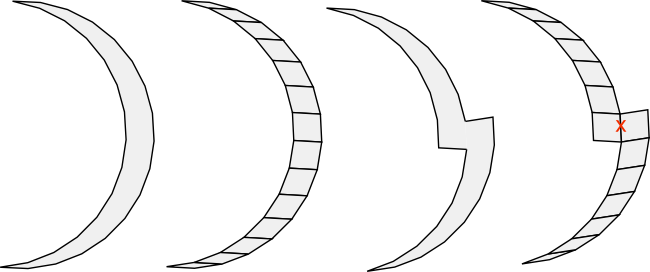In the first case, the crescent shaped polygon is a worm because it is made from rhombs that follow the edge criteria. However, the second polygon is not a worm because two of the rhombs that make it up are joined at an incorrect non-parallel edge (marked with the red x).

Any worm can trivially be decomposed into rhombs by adding new edges that join opposite vertices.

### Zonagons

A zonagon is a polygon which satisfies the following criteria:

• it is convex
• each edge has the same length
• each edge a has exactly one matching parallel edge b (a != b)

Zonagons always have an even number of sides. Any regular polygon with an even number of sides is a zonagon, but not all zonagons are regular. For example, this irregular decagon is a zonagon: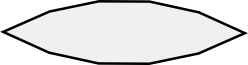Rhombs are zonagons with 4 sides. In some ways a zonagon is a generalization of the idea of a rhomb to polygons that have more than 4 sides.

I won't prove it here, but zonagons always meet the KSK criteria and hence can always be decomposed into rhombs.

Note: some older definitions of zonagons require only that each parallel pair of edges be the same length. This definition would make a zonagon a generalisation of a parallelogram rather than a rhomb. However, the trend recently is to require that all the edges be the same length and that is the definition that I'm using here.

### The KSK algorithm

Although the KSK criteria for determining whether a polygon can be decomposed into rhombs are a bit technical, the actual algorithm is remarkably simple. It uses a classic recursive divide-and-conquer approach. The polygon to be decomposed is split into two smaller polygons, both of which also satisfy the KSK criteria. The algorithm can then be applied recursively on the smaller polygons until there is nothing left but rhombs.

Let's apply the algorithm to the 30-gon. This has an even number of sides and therefore is a zonagon. Like all zonagons, it satisfies the KSK criteria and so can be decomposed into rhombs.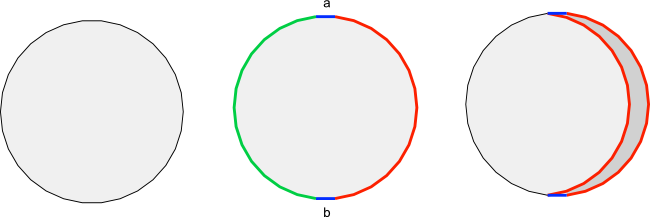The algorithm requires a matching function which takes the polygon to be decomposed and returns two parallel edges a and b (shown in blue in the diagram above) and a direction. The direction can be clockwise or counterclockwise and is used to generate a list of all the edges between a and b. If the direction is clockwise, the edges between would be those shown in red in the diagram above. If the direction is counterclockwise, the edges between would be those shown in green in the diagram above.

(Note: if we apply the KSK algorithm to a zonagon, the parallel edge b is always uniquely determined by a because zonagons always have exactly one other parallel edge. This is not necessarily true for concave polygons. Nevertheless Kenyon proves that regardless of the polygon being decomposed, given an edge a there is always exactly one other edge b that must be selected by all matching functions if the decomposition is to succeed.)

Let's assume that the matching function returns a direction of clockwise. We then select the red edges as those between the blue edges a and b. Make a copy of the red edges and translate the copy so that it is connected to the beginning of edge a and the end of edge b. The amazing part of the algorithm is that this edge translation always works! It works precisely because edges a and b are parallel and have the same length.

This splits the previous polygon into two. One of these new polygons is always a worm, and we will ignore that for now. We can now apply the algorithm recursively to the other polygon (the residual polygon) which has two fewer edges than the original polygon. If the starting polygon is a zonagon, so is the residual polygon. We continue applying the algorithm to the residual polygon until there is nothing left but worms. It is then a trivial last step to split the worms into rhombs.

In my implementation of the KSK algorithm, I use a matching function that alternates the direction selected at each stage between clockwise and counterclockwise. This ensures that the residual polygon shrinks towards the centre of the original polygon, resulting in an aesthetically pleasing symmetry.

Many different matching functions are possible and return different decompositions of the original polygon. No formula is known that gives the number of ways that a 2n-sided regular polygon can be decomposed into rhombs, but curiously there is a simple formula to determine the number of rhombs. No matter how it is decomposed, a 2n-sided regular polygon is always divided into n(n-1)/2 rhombs. In the case of the 30-gon, this is 105 rhombs.

In general, a polygon with 2n sides requires n-2 stages to decompose. Here are the 13 stages for the 30-gon and the final result: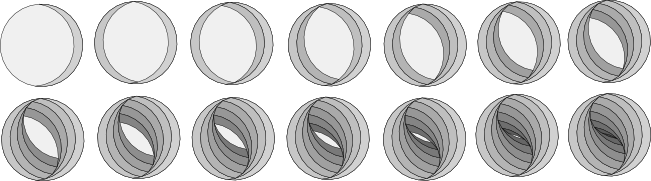Once the polygon is divided entirely into worms, it is a simple matter to split the worms into rhombs. The matching function I have used produces something like a yin and yang symbol.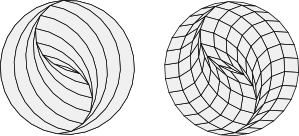It turns out that decomposing zonagons (including 2n-sided regular polygons) into rhombs is remarkably simple.

The situation becomes slightly more complex (and quite a bit more interesting) when we look at the extended algorithm to decompose regular polygons into rhombs and smaller regular polygons. I'll discuss that in the next section.